# ISEE Middle Level Math : How to find percentage

## Example Questions

### Example Question #71 : Percentage

What is the decimal equivalent of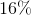?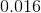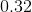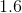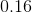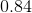Explanation:

To solve, divide by one hundred: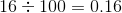Answer:### Example Question #72 : Percentage

What is the decimal equivalent of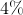?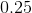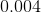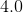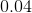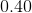Explanation:

To solve, divide by one hundred: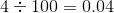Answer:### Example Question #73 : Percentage

What is the decimal equivalent of?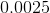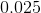Explanation:

To solve, divide by one hundred: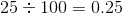Answer:### Example Question #74 : Percentage

What is the equivalent of%?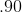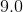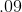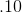Explanation:

Divide: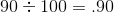Answer:### Example Question #71 : How To Find Percentage

What is the equivalent of%?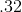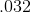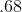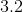Explanation:

Divide: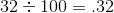Answer:### Example Question #76 : Percentage

What is the equivalent of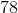%?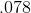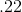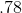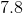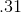Explanation:

Divide: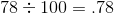Answer:### Example Question #77 : Percentage

What is the equivalent of%?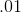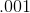Explanation:

Divide: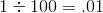### Example Question #78 : Percentage

What is the equivalent of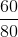?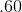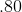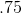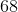Explanation:

Divide: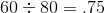Answer:### Example Question #781 : Hspt Mathematics

What is the equivalent of%?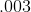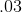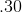Explanation:

Divide: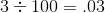Answer:### Example Question #80 : Percentage

Find the decimal equivalent of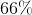.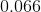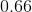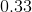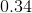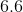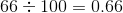Answer: